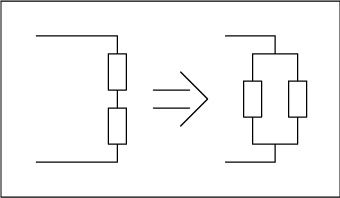# Measuring Differential Impedances with a Two-Port Network Analyzer

### Abstract

Many RF ICs use differential inputs and outputs. This article describes how a two-port network analyzer is used to measure differential impedance. Gamma is calculated from the measured S-parameters. The transformation between series and parallel models is given.

For noise suppression reasons it is very common in RF and microwave circuits to find differential input and output ports. Unfortunately, the impedance measurement of differential circuits is not straightforward with common RF test equipment.

The following measurement method will prevent the use of baluns and transformers, which introduce errors and will also provide a more accurate and repeatable measurement method of the differential impedance.

This example will use a mixer, which uses a single-ended input and a differential or balance output.Figure 1.

Measurement Procedure:

1. The first step is to do a full two-port calibration on the network analyzer
2. Activate the mixer to its normal operating conditions
3. Measure all parameters S11, S12, S21, and S22
4. Finally, use the equation below to convert the differential measurement to a single-ended resultFrom this equation, calculate the real and imaginary components to arrive at a parallel equivalent circuit:Figure 2. Parallel equivalent circuit of the series circuit.The fastest way to prove that this method actually works is by performing a simulation of a one-port differential circuit with two measurement ports single-ended and one measurement port differential. Calculate Γ d for circuit #1 and compare to Γ of circuit #2. The result should be the same.Figure 3.

The derivation of Γ d can be found in the January 1999 edition of RF Design Journal.